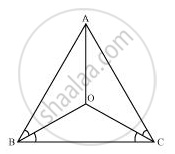# In an isosceles triangle ABC, with AB = AC, the bisectors of ∠B and ∠C intersect each other at O. Join A to O. Show that: (i) OB = OC (ii) AO bisects ∠A - Mathematics

In an isosceles triangle ABC, with AB = AC, the bisectors of ∠B and ∠C intersect each other at O. Join A to O. Show that: (i) OB = OC (ii) AO bisects ∠A

#### Solution(i) It is given that in triangle ABC, AB = AC

⇒ ∠ACB = ∠ABC (Angles opposite to equal sides of a triangle are equal)

⇒ 1/2∠ACB = 1/2∠ABC

⇒ ∠OCB = ∠OBC

⇒ OB = OC (Sides opposite to equal angles of a triangle are also equal)

(ii) In ΔOAB and ΔOAC,

AO =AO (Common)

AB = AC (Given)

OB = OC (Proved above)

Therefore, ΔOAB ≅ ΔOAC (By SSS congruence rule)

⇒ ∠BAO = ∠CAO (CPCT)

⇒ AO bisects ∠A.

Concept: Properties of a Triangle
Is there an error in this question or solution?

#### APPEARS IN

NCERT Class 9 Maths
Chapter 7 Triangles
Exercise 7.2 | Q 1 | Page 123